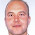# Learning Clojure

## Wednesday, August 18, 2010

### A Special Squarish Age

Microsoft Research published some problems for their 15th anniversary, some of which are quite tricky.
This one isn't, but I liked the program I wrote to solve it because it demonstrates how nice lazy streams are.

```
;; Microsoft Research 15th Anniversary Problem: A special squarish age.

;; A number is squarish if it is the product of two consecutive integers.
;; e.g. 6 is squarish because it is the product of 2 and 3

;; A colleague claims that his age is squarish, and furthermore, that his last squarish birthday was a squarish number of years ago.
;; What age could he be?

(def squarish (map (fn[[x y]] (* x y)) (partition 2 1 (iterate inc 0))))
(def squarishdiffs (map - (drop 1 squarish) squarish))

(defn find-in-increasing-seq [x seq]
(cond (empty? seq) false
(< (first seq) x) (recur x (rest seq))
(= (first seq) x) true
(> (first seq) x) false))

(defn squarish? [x] (find-in-increasing-seq x squarish))

(def possible-ages (mapcat
(fn [s d] (if (squarish? d) (list s) '()))
(drop 1 squarish)
squarishdiffs))

(take 10 possible-ages)

;; He's probably 42. 12 is a bit young for a colleague, 110 is pushing it.```

1.Nice!
Can we redefine find-in-increasing-seq as follow:
(defn find-in-increasing-seq [x seq]
(some #(= x) seq))

2.Tzach, you could if the sequence was finite. (I'm assuming you mean #(= % x) rather than #(= x), which is always true.)

With an infinite sequence, how will it know to stop searching if it doesn't find x after the first 1000000 entries?